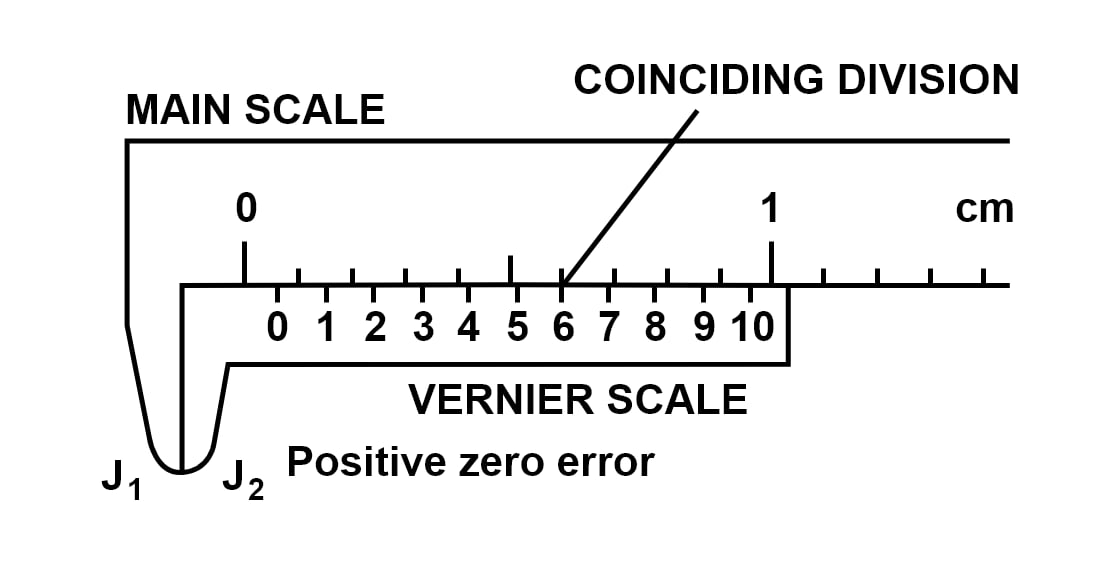# A vernier calliper has a zero error + 0.06 cm. Draw a neat digram to represent it

Solution

Below diagram shows a vernier callipers with a zero error of +0.06 cm.The least count of the vernier callipers shown in the diagram is 0.01 cm and the 6th division of the vernier scale, coincides with a main scale division.

Therefore,

Zero Error=6×L.C.
Zero Error=6×0.01cm
⇒Zero Error=0.06cm

• 0
What are you looking for?